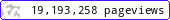•••••MadAsMaths.com :: I.Y.G.B. Practice Papers :: A Level Practice Papers 2019 Spec :: MMS Practice Papers

I.Y.G.B. MMS PRACTICE PAPERS

These I.Y.G.B. practice papers mostly follow the Pearson/Edexcel Syllabus introduced for teaching from 2017.

Some modules will be first examined in 2018 and some in 2019.

• Papers A to R have standard difficulty with later papers usually more difficult.
• Papers S and T are extremely hard.
• Papers U to Z are hard.

All papers have model solutions.

IMPORTANT

In the actual exam the Statistics/Mechanics paper will be out of 100 marks.

These practice papers are out of 150 marks which makes the coverage of the topics exhaustive.

 mms_a.pdfmms_a_solutions.pdfmms_b.pdfmms_b_solutions.pdfmms_c.pdfmms_c_solutions.pdfmms_d.pdfmms_d_solutions.pdfmms_e.pdfmms_e_solutions.pdfmms_f.pdfmms_f_solutions.pdfmms_g.pdfmms_g_solutions.pdfmms_h.pdfmms_h_solutions.pdfmms_i.pdfmms_i_solutions.pdfmms_j.pdfmms_j_solutions.pdfmms_k.pdfmms_k_solutions.pdfmms_l.pdfmms_l_solutions.pdfmms_m.pdfmms_m_solutions.pdfmms_n.pdfmms_n_solutions.pdfmms_o.pdfmms_o_solutions.pdfmms_p.pdfmms_p_solutions.pdfmms_q.pdfmms_q_solutions.pdfmms_r.pdfmms_r_solutions.pdfmms_s.pdfmms_s_solutions.pdfmms_t.pdfmms_t_solutions.pdfmms_u.pdfmms_u_solutions.pdfmms_v.pdfmms_v_solutions.pdfmms_w.pdfmms_w_solutions.pdfmms_x.pdfmms_x_solutions.pdfmms_y.pdfmms_y_solutions.pdfmms_z.pdfmms_z_solutions.pdf